Related Articles

# Introduction to Recurrent Neural Network

• Difficulty Level : Medium
• Last Updated : 03 Oct, 2018

Recurrent Neural Network(RNN) are a type of Neural Network where the output from previous step are fed as input to the current step. In traditional neural networks, all the inputs and outputs are independent of each other, but in cases like when it is required to predict the next word of a sentence, the previous words are required and hence there is a need to remember the previous words. Thus RNN came into existence, which solved this issue with the help of a Hidden Layer. The main and most important feature of RNN is Hidden state, which remembers some information about a sequence.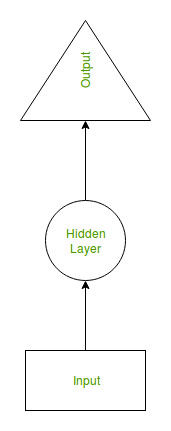RNN have a “memory” which remembers all information about what has been calculated. It uses the same parameters for each input as it performs the same task on all the inputs or hidden layers to produce the output. This reduces the complexity of parameters, unlike other neural networks.

### How RNN works

The working of a RNN can be understood with the help of below example:

Example:

Suppose there is a deeper network with one input layer, three hidden layers and one output layer. Then like other neural networks, each hidden layer will have its own set of weights and biases, let’s say, for hidden layer 1 the weights and biases are (w1, b1), (w2, b2) for second hidden layer and (w3, b3) for third hidden layer. This means that each of these layers are independent of each other, i.e. they do not memorize the previous outputs.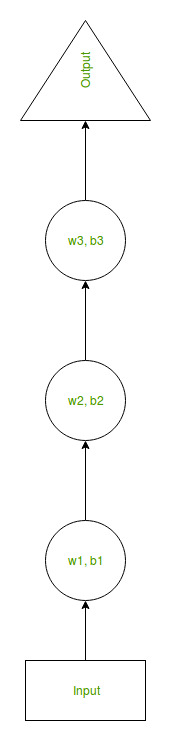Now the RNN will do the following:

• RNN converts the independent activations into dependent activations by providing the same weights and biases to all the layers, thus reducing the complexity of increasing parameters and memorizing each previous outputs by giving each output as input to the next hidden layer.
• Hence these three layers can be joined together such that the weights and bias of all the hidden layers is the same, into a single recurrent layer.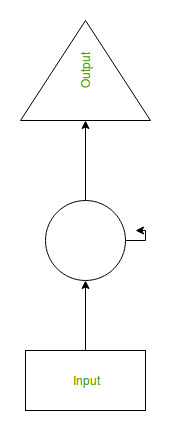• Formula for calculating current state: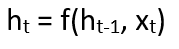where:

```ht -> current state
ht-1 -> previous state
xt -> input state
```
• Formula for applying Activation function(tanh):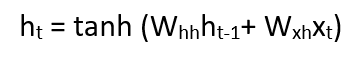where:

```whh -> weight at recurrent neuron
wxh -> weight at input neuron
```
• Formula for calculating output: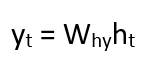```Yt -> output
Why -> weight at output layer
```

### Training through RNN

1. A single time step of the input is provided to the network.
2. Then calculate its current state using set of current input and the previous state.
3. The current ht becomes ht-1 for the next time step.
4. One can go as many time steps according to the problem and join the information from all the previous states.
5. Once all the time steps are completed the final current state is used to calculate the output.
6. The output is then compared to the actual output i.e the target output and the error is generated.
7. The error is then back-propagated to the network to update the weights and hence the network (RNN) is trained.

### Advantages of Recurrent Neural Network

1. An RNN remembers each and every information through time. It is useful in time series prediction only because of the feature to remember previous inputs as well. This is called Long Short Term Memory.
2. Recurrent neural network are even used with convolutional layers to extend the effective pixel neighborhood.